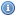# Linear & Abstract Algebra Books, Documents, and Lectures

 Title Year DescriptionA Course In Commutative Algebra 2003Commutative algebra is the theoretical foundation of algebraic geometry and algebraic number theory. Assuming as prerequisite a standard graduate algebra course, the author attempts to reach an advanced level quickly and efficiently.A Course in Universal Algebra 1981This book offers an excellent introduction to the subject. This text is not intended to be encyclopedic; rather, a few themes central to universal algebra have been developed sufficiently to bring the reader to the brink of current research.Abstract Algebra Online 2004This site contains many of the definitions and theorems from the area of mathematics generally called abstract algebra. It is intended for undergraduate students taking an abstract algebra class at the junior/senior level.Abstract Algebra: The Basic Graduate Year 2002This is a student-oriented text covering the standard first year graduate course in algebra. Solutions to all problems are included and some of the reasoning is informal.AlgebraA set of online notes for an Algebra course taught at Lamar University.Algebra: Abstract and Concrete 2006This text provides a thorough introduction to "modern'' or "abstract'' algebra at the upper-level undergraduates and beginning graduate level. The book addresses : groups, rings, fields, and linear algebra, with symmetry as a unifying theme.Application of Linear AlgebraThese notes hope to introduce linear algebra packages LINPACK, EISPACK and LAPACK, as well as MATLAB, MAPLE, MATHCAD and MATHEMATICA as they relate to linear algegbra.Elementary Linear Algebra 1991This book is an introduction to linear algebra, based on lectures given over 17 years.Elements of Abstract and Linear Algebra 2004This text is written with the conviction that it is more effective to teach abstract and linear algebra as one coherent discipline rather than as two separate ones.Lecture Notes on Numerical Linear Algebra 2001A brief set of notes on numerical linear algebraLinear AlgebraA linear algebra textbook written for undergraduates. Topics covered included gaussian reduction, vector spaces, linear maps, determinants, and eigenvalues and eigenvectors. The book’s presentation emphasizes motivation and naturalness.Linear Algebra (Lamar Univeristy)A set of online notes for a Linear Algebra course taught Lamar University.Linear Algebra - An Introduction to Linear Algebra for Pre-Calculus Students 2000This book is an introduction to linear algebra for pre-calculus students. It is a stand-alone unit in the sense that no prior knowledge of matrices is assumed.Linear Algebra Lecture Notes 2003These pages are lecture notes for a Linear Algebra course at the University of Queensland.Linear Algebra Lecture Notes - William Chen (Macquarie University)Lecture notes for a first linear algebra course compiled over 25 years.Linear Methods of Applied Mathematics 2000The book is targeted at engineering students who have had two years of calculus, introductory linear algebra, and introductory ordinary differential equations.Structure and Representations of Jordan Algebras 1968The purpoe of this book is to give a comprehensive account of the structure and representation of Jordan algebras over a field of characteristic not two.Templates for the Solution of Linear SystemsA book that provides templates: Description of an algorithm, abstracting away from implementational details. A template is a description of a general algorithm rather than the executable object code or the source code.